# Test: Kirchhoff’s Current & Voltage Law

## 20 Questions MCQ Test Network Theory (Electric Circuits) | Test: Kirchhoff’s Current & Voltage Law

Description
Attempt Test: Kirchhoff’s Current & Voltage Law | 20 questions in 20 minutes | Mock test for Electrical Engineering (EE) preparation | Free important questions MCQ to study Network Theory (Electric Circuits) for Electrical Engineering (EE) Exam | Download free PDF with solutions
QUESTION: 1

### Find the value of v if v1=20V. Find the value of v if v1=20V and value of current source is 6A.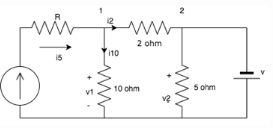Solution:

The current through the 10 ohm resistor=v1/10=2A.Applying KCL at node 1: i5=i10+i2. i2=6-2=4A.
Thus the drop in the 2 ohm resistor= 4×2=8V.
v1=20V; hence v2=20-v across 2 ohm resistor=20-8=12V
v2=v since they are connected in parallel.
v=12V.

QUESTION: 2

### Calculate the current A.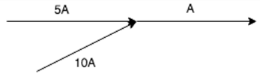Solution:

KCl states that the total current leaving the junction is equal to the current entering it. In this case, the current entering the junction is 5A+10A=15A.

QUESTION: 3

### Calculate the current across the 20 ohm resistor.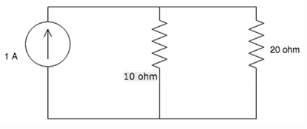Solution:

Assume lower terminal of 20 ohm at 0V and upper terminal at V volt and applying KCL, we get V/10 +V/20 =1. V=20/3V So current through 20 ohm = V/20 = (20/3)/20 =1/3=0.33V.

QUESTION: 4

Calculate the value of I3, if I1= 2A and I2=3A.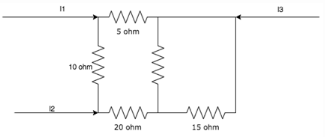Solution:

According to KCl, I1+I2+I3=0. Hence I3=-(I1+I2)=-5A.

QUESTION: 5

Find the value of i2, i4 and i5 if i1=3A, i3=1A and i6=1A.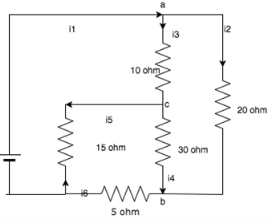Solution:

At junction a: i1-i3-i2=0. i2=2A.
At junction b: i4+i2-i6=0. i4=-1A.
At junction c: i3-i5+i4=0. i5=2A.

QUESTION: 6

What is the value of current if a 50C charge flows in a conductor over a period of 5 seconds?

Solution:

Current=Charge/Time. Here charge = 50c and time = 5seconds, so current = 50/5 = 10A.

QUESTION: 7

KCL deals with the conservation of?

Solution:

KCL states that the amount of charge entering a junction is equal to the amount of charge leaving it, hence it is the conservation of charge.

QUESTION: 8

KCL is applied at _________

Solution:

KCL states that the amount of charge leaving a node is equal to the amount of charge entering it, hence it is applied at nodes.

QUESTION: 9

KCL can be applied for __________

Solution:

KCL is applied for different nodes of a network whether it is planar or non-planar.

QUESTION: 10

What is the value of the current I?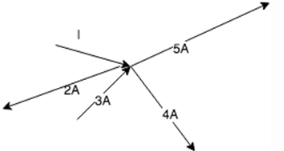Solution:

At the junction, I-2+3-4-5=0. Hence I=8A.

QUESTION: 11

Calculate the value of V1 and V2.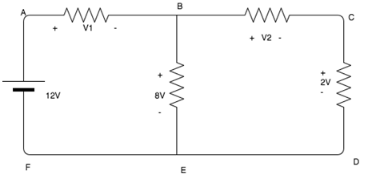Solution:

Using KVL, 12-V1-8=0. V1= 4V.
8-V2-2=0. V2=6V.

QUESTION: 12

KCL deals with the conservation of?

Solution:

KCL states that the amount of charge entering a junction is equal to the amount of charge leaving it, hence it is the conservation of charge.

QUESTION: 13

Calculate the voltage across the 10 ohm resistor.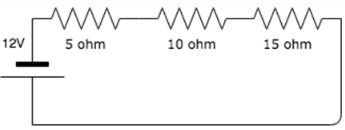Solution:

Using voltage divider rule, V=10*12/30=4V.

QUESTION: 14

Find the value of the currents I1 and I2.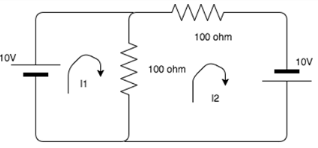Solution:

Using KVL, the matrix to find the loop currents are:
MATRIX 10 -100*i1 + 100*i2= 0
MATRIX  10-200*i2 + 100i1 = 0
I1= 0.3, I2=0.2

QUESTION: 15

The sum of the voltages over any closed loop is equal to __________

Solution:

According to KVL, the sum of the voltage over any closed loop is equal to 0.

QUESTION: 16

What is the basic law that has to be followed in order to analyze ant circuit?

Solution:

Kirchhoff’s laws, namely Kirchhoff’s Current Law and Kirchhoff’s Voltage law are the basic laws in order to analyze a circuit.

QUESTION: 17

Every____________ is a ____________ but every __________ is not a __________

Solution:

According to Kirchhoff’s Voltage Law, Every mesh is a loop but every loop is not a mesh.

QUESTION: 18

Solve for I.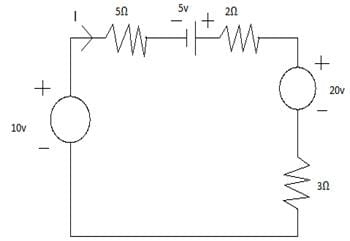Solution:

Veq = 10 + 5 -20 = -5u
Req = 5 + 2 + 3 = 10Ω
I = V/R = -5/10 = -0.5A.

QUESTION: 19

Calculate VAB.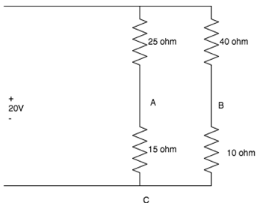Solution:

For branch A: VAC=15*20/(25+15)=7.5V
For branch B: VBC= 10*20/(10+40)=4V
Applying KVL to loop ABC:
VAB+VBC+VCA=0
VAB=3.5V.

QUESTION: 20

KVL is applied in ____________

Solution:

Mesh analysis helps us to utilize the different voltages in the circuit as well as the IR products in the circuit which is nothing but KVL.Use Code STAYHOME200 and get INR 200 additional OFF Use Coupon Code